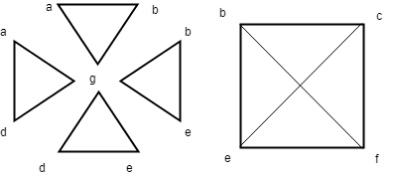# UGC NET COMPUTER SCIENCE SOLVED PAPERS 2014-16 - UGC NET Computer Science Paper 2 JULY 2016

>>>>>>>>UGC NET Computer Science Paper 2 JULY 2016

• A

10• B

15• C

25• D

30• A

14, 14• B

16, 14• C

16, 4• D

14, 4• Option : C
• Explanation :
Spanning trees in complete graph is equal to n(n-2)(where n is no of sides or regularity in complete graph). So, spanning trees in complete graph K4 will be 4(4 - 2). i.e. 42 = 16. Spanning trees in a bipartite graph Km,n is equal to m(n-1) * n(m-1). So, spanning trees in K2,2 will be 2(2-1) * 2(2-1). i.e. 21 * 21.= 4. So, option (C) is correct.

• A

3/4• B

2/3• C

1/2• D

1/3• Option : B
• Explanation :
There are BB RR and BR, total outcome is 3, probability that the opposite side is the same color as the one side we observed: It is clear that BB and RR will show the same color on opposite side, so favorable outcome will be 2. Probability will be favorable outcome / total outcome i.e. 2 / 3. So, option (B) is correct.

• A

2• B

4• C

5• D

6• Option : C
• Explanation :Total 5 clique will be there. So, option (C) is correct.